﻿ 20-sim webhelp > Modeling Tutorial > Bond Graphs > From Bond Graph to Equations

# From Bond Graph to Equations

With a causal bond graph model, equivalent dynamic equations can easily be derived. To create the dynamic equations, the following steps have to be performed.

 • Denote for every bond its effort and flow pair. You can use names that are obvious or methodically use numbers. Some examples are shown at the right.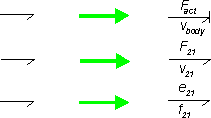• Replace elements by corresponding dynamic equations. Use the correct effort and flow description which can be found in the element tables. As an example descriptions for the R-element are given.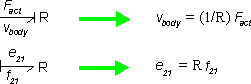• Replace the junctions by the correct equations. For 0 junctions the efforts are equal. All flows of the bonds pointing towards the 0 junction should be added and all flows of the bonds pointing from the 0 junction should be subtracted. For 1 junctions the flows are equal. All efforts of the bonds pointing towards the 0 junction should be added and all efforts of the bonds pointing from the 0 junction should be subtracted. Some examples are shown at the right.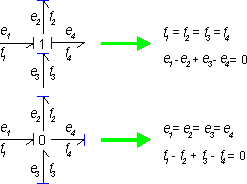• Rearrange the equations by removing redundant variables.

## Example

As an example in the figure below a causal bond graph model is shown. We will derive the set of dynamic equations out of this model.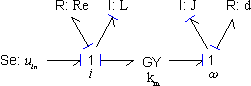1 Denote all efforts and flows. This is shown below: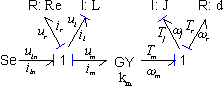2 Write the dynamic equations of the elements and junctions:

## equations

Se

uin = constant , iin = free

R

ur = Re ir

I

il = (1/L) int(ul)

1 junction

iin = ir = il = im

uin - ur - ul - um = 0

GY

um = km ωm

Tm = km im

I

Tj = (1/J) int(ωj)

R

Tr = d ωr

1 junction

ωm = ωj = ωr

Tm - Tl - Tr = 0

 3 Reduce the amount of equations. We replace the equalities of the 1 junctions: iin = ir = il = im= i and ωm = ωj = ωr= ω .

## equations

Se

uin = constant

R

ur = Re i

I

i = (1/L) int(ul)

1 junction

uin - ur - ul - um = 0

GY

um = km ω

Tm = km i

I

Tj = (1/J) int(ω)

R

Tr = d ω

1 junction

Tm - Tl - Tr = 0

The resulting dynamic equations can be used for simulation. 20-sim can automatically extract the dynamic equations out of a bond graph model. The equations can be shown using the Show Equations command or used in the Simulator for simulation.